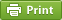Werner's Blog — Opinion, Analysis, Commentary
Bivariate Normal Random Numbers

Empirical economic work often involves simulations and drawing random numbers. Drawing random numbers from a multivariate distribution is increasingly becoming standardized in a variety of software packages. Stata, for example, has the drawnorm command which allows sampling from a multivariate normal distribution. SAS has the PROC SIMNORMAL procedure, as well as the RANDNORMAL function in SAS/IML. The R statistical platform has the mvrnorm function. Many of these packages cover other types of distributions in addition to the normal distribution.

The aforementioned functions are highly effective tools. Once in a while, it may also be beneficial to simply draw from a bivariate normal distribution without relying on one of the aforementioned packages. There are actually two ways of doing this on the quick. The first method involves the conditional distribution of a random variable $$X_2$$ given $$X_1$$. Therefore, a bivariate normal distribution can be simulated by drawing a random variable from the marginal normal distribution and then drawing a second random variable from the conditional normal distribution. The C++11 code fragment below shows how to do this, using the standard library random generator for normal distributions. We start with parameters $$\mu_1$$ and $$\sigma_1$$ for the mean and standard deviation for variable 1, parameters $$\mu_2$$ and $$\sigma_2$$ for the mean and standard deviation for variable 2, and $$\rho\in[-1,+1]$$ as the correlation coefficient. The code fragment populates vectors v1 and v2.

// bivariate random normal variables void rbvnorm(std::vector &v1, std::vector &v2, double mu1,double mu2, double sigma1,double sigma2,double rho) { std::default_random_engine generator; std::normal_distribution standardnormal(0.0,1.0); double lambda=(sigma2/sigma1)*rho; double nu=sqrt((1-rho*rho)*sigma2*sigma2); for (int i=0;i<v1.size();++i) { double x1=v1[i]=mu1+sigma1*standardnormal(generator); v2[i]=mu2+lambda*(x1-mu1)+nu*standardnormal(generator); } }

The code above is simple and quick. There is an alternative approach that underlies the method used for multivariate normal distributions. It requires the spectral decomposition of the variance-covariance matrix $V=\left[\begin{array}{cc}\sigma_1^2&\rho\sigma_1\sigma_2\\ \rho\sigma_1\sigma_2&\sigma_2^2\end{array}\right]=U \Lambda U^\top$ where $$U$$ is the matrix of normalized eigenvectors corresponding to the diagonal matrix of eigenvalues $$\Lambda$$. To generate random bivariate normal variables $$\{x_1,x_2\}$$ we first generate two random standard normal variables $$\{z_1,z_2\}$$ and transform them through the matrix $$\Phi\equiv U\Lambda^{1/2}$$ to yield $\left[\begin{array}{c}x_1\\x_2\end{array}\right]= \left[\begin{array}{c}\mu_1\\ \mu_2\end{array}\right]+ \left[\begin{array}{cc} \phi_{11}&\phi_{12}\\ \phi_{21}&\phi_{22} \end{array}\right]\cdot \left[\begin{array}{c}z_1\\z_2\end{array}\right]$ The four elements of the $$\Phi$$ matrix can be calculated explicitly, as eigenvalues and normalized eigenvectors of a two-by-two matrix are not overly complicated. (N.B.: eigenvectors computed by mathematical applications are not normalized—made to be of length one—by default. In deriving the code fragment below, normalization is a crucial step.) The result is the code fragment below that requires the calculation of six auxiliary variables from the elements of the variance-covariance matrix. These six auxiliary variables (G, H, K, J, U, V) are then used to compute the elements of the transformation matrix $$\Phi$$. The loop to populate vectors v1 and v2 are then straight-forward. The eigenvalues $$\lambda_1$$ and $$\lambda_2$$ of the variance-covariance matrix are $\begin{array}{lll} \lambda_1=(G+H)/2& \quad G=\sigma_1^2+\sigma_2^2& \quad K=\rho\sigma_1\sigma_2\\ \lambda_2=(G-H)/2& \quad J=\sigma_2^2-\sigma_1^2& \quad H=\sqrt{J^2+4K^2} \end{array}$ Prior to normalization, the eigenvectors are given by $\left[\begin{array}{cc}2K/(J+H)&2K/(J-H)\\ 1&1\end{array}\right]$ Normalizing each column in this matrix to length 1 and post-multiplying the resulting matrix with the diagonal matrix with the square roots of the two eigenvalues then delivers the code below. It has a few more lines than the first version of code, but is perhaps slightly more elegant.

// bivariate random normal variables double sq(double x) { return(x*x); } void rbvnorm(std::vector &v1, std::vector &v2, double mu1,double mu2, double sigma1,double sigma2,double rho) { std::default_random_engine generator; std::normal_distribution standardnormal(0.0,1.0); double phi11,phi12,phi21,phi22; if (rho==0.0) { phi11=sigma1; phi22=sigma2; sigma12=sigma21=0.0; } else if (rho==1.0) { phi11=sigma1; phi21=sigma2; phi21=phi22=0.0; } else if (rho==-1.0) { phi11=sigma1; phi21=-sigma2; phi21=phi22=0.0; } else { double G = sq(sigma1)+sq(sigma2); double J = sq(sigma2)-sq(sigma1); double K = rho*sigma1*sigma2; double H = sqrt(sq(J)+4.0*sq(K)); double U = sqrt(2.0*(G+H)/(sq(H+J)+4.0*sq(K))); double V = sqrt(2.0*(G-H)/(sq(H-J)+4.0*sq(K))); phi11 = K*U; phi12 = -K*V; phi21 = (J+H)*U/2.0; phi22 = (H-J)*V/2.0; } for (int i=0;i<v1.size();++i) { double z1=standardnormal(generator); double z2=standardnormal(generator); v1[i]=mu1+phi11*z1+phi12*z2; v2[i]=mu2+phi21*z1+phi22*z2; } }

So is there any benefit in using the more complicated version? Other than the fun of solving the spectral decomposition explicitly for the bivariate case, there is actually some caution needed for that version. There are already three special cases that need to be filtered out: perfect positive and negative correlation, and zero correlation. In all three cases we have problems. When $$\rho=0$$ then $$K=0$$, and thus $$H=J$$ and therefore $$V$$ is not defined because the denominator is zero. Other problems arise at the boundaries when $$\rho$$ approaches -1 or +1, which may lead to numerical instability in the calculations. So all in all, the better choice is the simpler algorithm. There is then but one question: does it matter in which order one computes the two random variables? If one favours numerical stability, it makes sense to order the variables so that $$\sigma_1>\sigma_2$$ so that $$\lambda$$ remains small. This prevents small errors in either standard deviation from being magnified through $$\lambda$$. A slightly refined version of the above script would swap the variables so that the ordering of the standard deviations is optimal.

Posted on Sunday, March 3, 2019 at 07:30 — #Econometrics | #Software
Updated on Sunday, March 10, 2019Recent Blog Entries

Topics

Months

Subscribe to RSS feed© 2023  Prof. Werner Antweiler, University of British Columbia.